# Texas Go Math Grade 4 Lesson 7.4 Answer Key Multiply Using Expanded Form

Refer to our Texas Go Math Grade 4 Answer Key Pdf to score good marks in the exams. Test yourself by practicing the problems from Texas Go Math Grade 4 Lesson 7.4 Answer Key Multiply Using Expanded Form.

## Texas Go Math Grade 4 Lesson 7.4 Answer Key Multiply Using Expanded Form

Essential Question

How can you use expanded form to multiply a multidigit number by a 1-digit number?

Unlock the problem

Example 1 Use expanded form.

Multiply. 5 × 143
5 × 143 = 5 × (__________ + __________ + __________) Write 143 in expanded form.
= (5 × 100) + (__________ × __________) + (__________ × __________) Use the Distributive Property.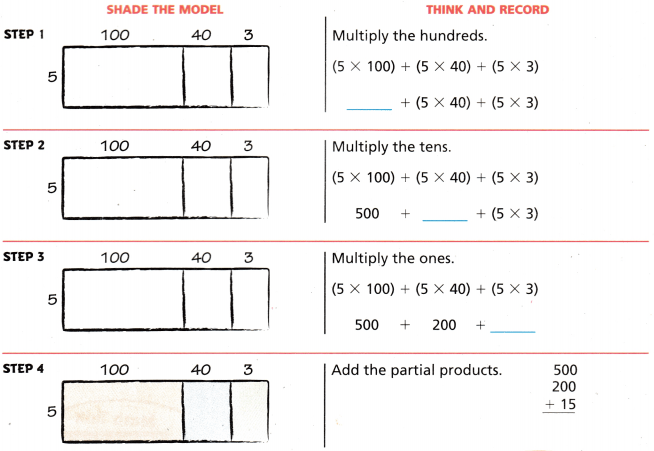So, 5 × 143 = __________.
5 × 143 = 715.

Explanation:
Here, 143 is between 100 and 200. So, 5 × 143 is between 5 × 100 = 500 and 5 × 200 = 1,000. Since 715 is between 500 and 1,000.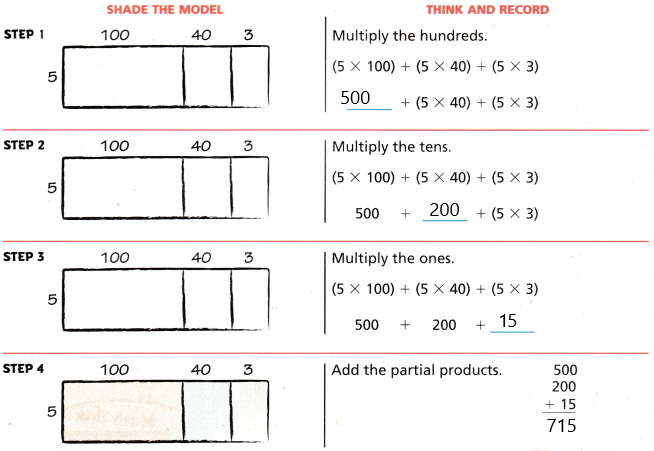So, 5 × 143 = 715.

Math Talk

Mathematical Processes
Here, 143 is between 100 and 200. So, 5 × 143 is between 5 × 100 = 500 and 5 × 200 = 1,000. Since 715 is between 500 and 1,000. So the answer is reasonable.

Example 2 Use expanded form.
The gift shop at the animal park orders 3 boxes of toy animals. Each box has 1,250 toy animals. How many toy animals does the shop order?
Multiply. 3 × 1,250

STEP 1
Write 1,250 in expanded form. Use the Distributive Property.
3 × 1,250 = 3 × (____________ + ____________ + ____________)
= (3 × 1,000) + (____________ × ____________) + (____________ × ____________)
3 × 1,250 = 3,750.

Explanation:
Here, the Distributive property means if we multiply a sum by a number is the same as multiplying each addend by the number and adding the products. So
3 × 1,250 = 3 × (1,000 + 200 + 50)
= (3 × 1,000)+ (3 × 200)+ (3 × 50)
= 3000+600+150
= 3,750.

STEP 2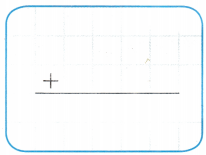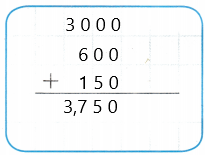So, the shop ordered 3,750 animals.

Share and Show

Question 1.
Find 4 × 213. Use expanded form.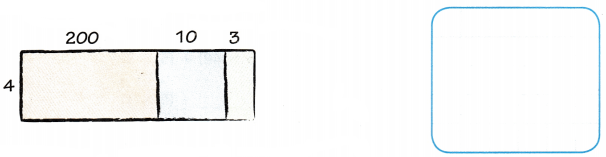4 × 213 = ____________ × (____________ + ____________ + ____________)
= (____________ × ____________) + (____________ × ____________) + (____________ × ____________) Use the Distributive Property
= ____________ + ____________ + ____________
= ____________

4 × 213 = 852.

Explanation:
Here, the Distributive property means if we multiply a sum by a number is the same as multiplying each addend by the number and adding the products. So
4 × 213 = 4 × (200+10+3)
= (4 × 200)+(4 × 10)+(4 × 3)
= 800+40+12
= 852.

Math Talk

Mathematical Processes
Explain how using an expanded form makes finding the product easier.
By expanded form, we can understand better and rightly read the higher digit numbers. By the place value, we can make sure that the digits are in the correct place.

Record the product. Use the expanded form to help.

Question 2.
4 × 59 = ___________
4 × 59 = 236.

Explanation:
Here, the Distributive property means multiplying a sum by a number is the same as multiplying each addend by the number and adding the products. So
4 × 59 = 4 × (50+9)
= (4 × 50) + (4 × 9)
= 200 + 36
= 236.

3 × 288 = ___________
3 × 288 = 864.

Explanation:
Here, the Distributive property means multiplying a sum by a number is the same as multiplying each addend by the number and adding the products. So
3 × 288 = 3 ×(200 + 80 +8)
= (3 × 200) + (3 × 80) + (3 × 8)
= 600+240+24
= 864.

Math Talk

Mathematical Processes
Explain how using an expanded form makes finding the product easier.
By expanded form, we can understand better and rightly read the higher digit numbers. And by the place value, we can make sure that the digits are in the correct place.

Problem-Solving

Use the table for 4-6.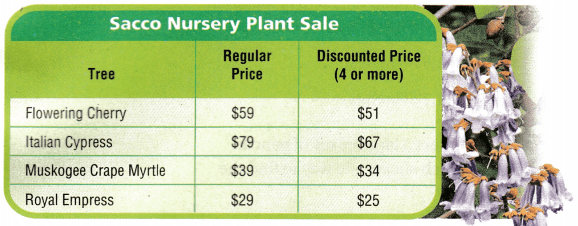Question 4.
What is the total cost of 3 Italian cypress trees?
The total cost of 3 Italian cypress trees is $237. Explanation: Given that the cost of Italian cypress is$79, so the total cost of 3 Italian cypress trees is $79×3 which is$237.

Question 5.
H.O.T. Multi-Step What’s the Error? Tanya says that the difference in the cost of 4 flowering cherry trees and 4 Muskogee crape myrtles is $80. Is she correct? Explain.Answer: Tanya is wrong. Explanation: Here, Tanya says that the difference in the cost of 4 flowering cherry trees and 4 Muskogee crape myrtles is$80. Given that the cost of 4 flowering cherry trees is $51 each which is$51×4 = $204 and 4 Muskogee crape myrtles is$34 each which is $34×4 =$136. And the difference between them is $204-$136 = $68. So Tanya is wrong. Question 6. H.O.T. Multi-Step What is the cost of 4 Royal Empress and 3 Muskogee Crape Myrtle? Answer: The total cost is$217.

Explanation:
Given that the cost of 4 Royal Empress is $25 each which is$25×4 = $100 and the cost of 3 Muskogee Crape Myrtle is$39×3 which is $117. So the total cost is$100+$117 which is$217.

Use Math Language What is the greatest possible product of a 2-digit number and a 1-digit number? Explain how you know.
The greatest possible product of a 2-digit number is 99 and a 1-digit number is 9. So the product is 99×9 which is 891.

Question 8.
Sara uses 5 rolls of tape to make a flower. Each roll has 344 inches of tape. How many inches of tape does Sara use to make the flower?
(A) 349 inches
(B) 1,504 inches
(C) 1,544 inches
(D) 1,720 inches
D.

Explanation:
Given that Sara uses 5 rolls of tape to make a flower and each roll has 344 inches of tape. So the number of inches of tape does Sara uses to make the flower is 5×344 which is 1,720 inches.

Question 9.
A worker at an office supply store orders 5 large boxes of pencils. Each box contains 6,120 pencils. Which shows one way to find the number of pencils the worker orders?
(A) (5 × 600) + (5 × 10) + (5 × 2)
(B) (5 × 6,000) + (5 × 100) + (5 × 2)
(C) (5 × 6,000) + (5 × 100) + (5 × 20)
(D) (5 × 6,000) + (5 × 10) + (5 × 2)
C.

Explanation:
Given that, a worker at an office supply store orders 5 large boxes of pencils and each box contains 6,120 pencils. So the one way to find the number of pencils the worker orders is (5 × 6,000) + (5 × 100) + (5 × 20).

Texas Go Math Grade 4 Lesson 7.4 Question 10.
Multi-Step A hotel has 128 rooms on each floor. There are 4 floors in all. If 334 of the rooms in the hotel have been cleaned, how many rooms still need to be cleaned?
(A) 178
(B) 206
(C) 384
(D) 512
A.

Explanations:
Given that a hotel has 128 rooms on each floor and there are 4 floors in all which is 128×4 which is 512 rooms. So if 334 of the rooms in the hotel have been cleaned, the number of rooms that still need to be cleaned is 512-334 which is 178 rooms.

TEXAS Test Prep

Question 11.
Which expression shows how to multiply 5 × 381 by using place value and expanded form?
(A) (5 × 3) + (5 × 8) + (5 × 1)
(B) (5 × 300) + (5 × 80) + (5 × 100)
(C) (5 × 300) + (5 × 80) + (5 × 1)
(D) (5 × 300) + (5 × 80) + (5 × 10)
C.

Explanation:
Given that 5 × 381, so by using place value and expanded form the expression will be
(5 × 300) + (5 × 80) + (5 × 1)

### Texas Go Math Grade 4 Lesson 7.4 Homework and Practice Answer Key

Record the product. Use expanded form to help.

Question 1.
5 × 73 = _____________
5 × 73 = 365.

Explanation:
Here, the Distributive property means if we multiply a sum by a number is the same as multiplying each addend by the number and adding the products. So
5 × 73 = 5 × (70+3)
= (5 × 70)+(5 × 3)
= 350+15
= 365.

Question 2.
6 × 24 = _____________
6 × 24 = 144.

Explanation:
Here, the Distributive property means if we multiply a sum by a number is the same as multiplying each addend by the number and adding the products. So
6 × 24 = 6 ×(20+4)
= (6 × 20)+(6 × 4)
= 120+24
= 144.

Problem Solving

Use the table for 3-6.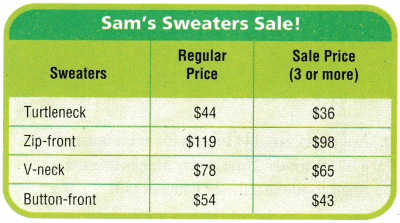Go Math 4th Grade Answer Key Practice and Homework Lesson 7.4 Question 3.
What is the cost of 2 v-neck sweaters?
The cost of 2 v-neck sweaters is $156. Explanation: Given that the cost of a v-neck sweater is$78, the cost of 2 v-neck sweaters is 2×$78 which is$156.

Question 4.
What is the cost of 5 button-front sweaters?
The cost of 5 button-front sweaters is $215. Explanation: Given that the cost of 5 button-front sweaters is$43 each, so $43×5 which is$215.

Question 5.
What is the cost of 4 v-neck sweaters and 2 zip-front sweaters?
The total cost is $498. Explanation: Given that the cost 4 v-neck sweaters is$65 each which is $65×4 =$260 and 2 zip-front sweaters is $119 each which is$119×2 = $238. So the total cost is$498.

Question 6.
Ben wants to buy 3 zip-front sweaters and 3 turtleneck sweaters. He says he will pay $225 more for the zip-front sweaters than for the turtleneck sweaters. Is he correct? Explain. Answer: Ben is wrong. Explanation: Here, Ben wants to buy 3 zip-front sweaters which costs$98 each which is $98×3 =$294 and the he wants to buy 3 turtleneck sweaters which costs $36 each which is$36×3 = $108. So the difference between them is 294-108 which is$186. So Ben is wrong.

Question 7.
What is the greatest possible product of a 3-digit number and a 1-digit number? Explain how you know.
The greatest possible product is 8,991.

Explanation:
The greatest 3-digit number is 999 and a 1-digit number is 9, so the greatest product is 999×9 which is 8,991.

Lesson Check

Question 8.
A jeweler has 36 inches of silver chain. She needs 5 times that much to make some necklaces. How much silver chain does the jeweler need to make her necklaces?
(A) 150 inches
(B) 41 inches
(C) 90 inches
(D) 180 inches
D.

Explanation:
Given that a jeweler has 36 inches of silver chain and she needs 5 times that much to make some necklaces. So the number of silver chain does the jeweler need to make her necklaces is 36×5 which is 180 inches.

Practice and Homework Lesson 7.4 Answer Key Question 9.
Gretchen walks her dog 3 times a day. Each time she walks the dog, she walks 1,760 yards. How many yards does she walk her dog in 1 day?
(A) 528 yards
(B) 3,390 yards
(C) 5,280 yards
(D) 3,228 yards
C.

Explanation:
Given that Gretchen walks her dog 3 times a day and each time she walks the dog, she walks 1,760 yards. So the number of yards does she walk her dog in 1 day is 1,760×3 which is 5,280 yards.

Question 10.
Angela needs 144 blocks for each layer of a block tower. How many blocks does she need to make a tower 8 layers tall?
(A) 1,152
(B) 720
(C) 1,064
(D) 1048
A.

Explanation:
Given that Angela needs 144 blocks for each layer of a block tower and the number of blocks does she need to make a tower 8 layers tall is 144×8 which is 1,152 blocks.

Question 11.
Which expression shows how to multiply 9 × 856 using place value and expanded form?
(A) (9 × 800) + (9 × 50) + (9 × 6)
(B) (9 × 8) + (9 × 5) +(9 × 6)
(C) (8 × 90) + (5 × 90) (6 × 9o)
(D) (9 × 80) +(9 × 50) + (9 × 60)
A.

Explanation:
Given that 9 × 856, so by place value and expanded form the expression is (9 × 800) + (9 × 50) + (9 × 6).

Question 12.
Multi-Step Jennifer bought 4 packages of tacks. There are 48 tacks in a package. She used 160 of the tacks to put up posters. How many tacks does she have left?
(A) 12
(B) 320
(C) 32
(D) 120
C.

Explanation:
Given that Jennifer bought 4 packages of tacks there are 48 tacks in a package which is 48×4 which is 192 and she used 160 of the tacks to put up posters. So the number of tacks she has left is 192-160 which is 32.

Texas Go Math Grade 4 Volume 1 Pdf Lesson 7.4 Question 13.
Jamal is making 8 wooden rails. Each rail is 42 inches long. How many inches of board does Jamal need to make all the rails?
(A) 238 inches
(B) 336 inches
(C) 342 inches
(D) 34 inches